Pythagoras Theorem

Chapter 6 Class 7 Triangle and its Properties
Concept wise

For a right angled triangle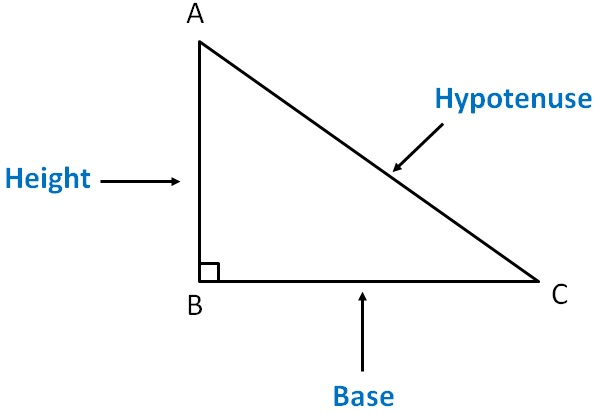Side opposite to right angle is Hypotenuse

Side adjacent to right angle are Base & height

Note :

Here, we can also take AB as base & BC as height.

It does not change our answer

Let   AB = a

BC = b

AC = c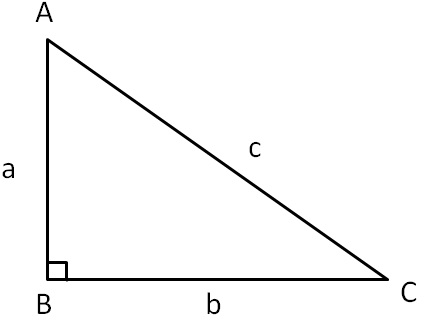Pythagoras theorem says that

Square of hypotenuse = Sum of square of other two sides

a 2 + b 2 = c 2

There are a lot of interesting things that we can do with Pythagoras theorem

Like

Let’s do some questions

Find x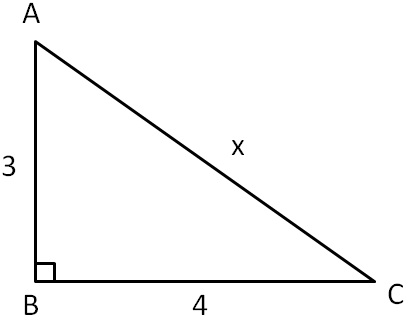Here,

∆ABC is a right angled triangle, right angled at B

And,

AB = 3, BC = 4, AC = x

By Pythagoras Theorem

AC 2 = AB 2 + BC 2

x 2 = 3 2 + 4 2

x 2 = 9 + 16

x 2 = 25

x 2 = 5 2

Cancelling squares

x = 5

Therefore, x = 5

Find x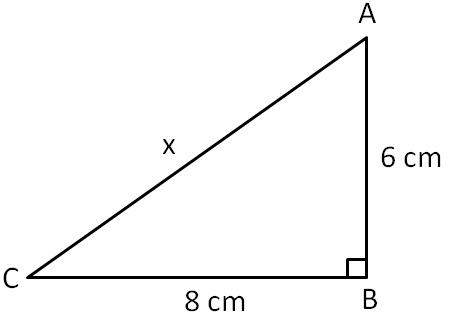Here,

∆ABC is a right angled triangle, right angled at B

And,

AB = 6 cm, BC = 8 cm, AC = x

By Pythagoras Theorem

AC 2 = AB 2 + BC 2

x 2 = 6 2 + 8 2

x 2 = 36 + 64

x 2 = 100

x 2 = 10 2

Cancelling squares

x = 10 cm

Therefore, x = 10 cm

Find x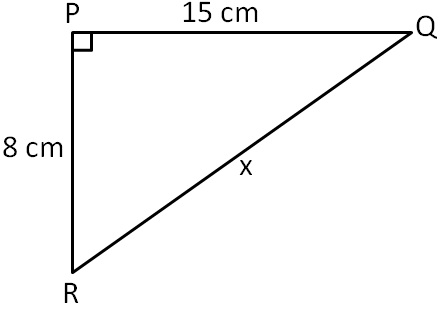Here,

∆PQR is a right angled triangle, right angled at P

And,

PR = 8 cm, PQ = 15 cm, QR = x

By Pythagoras Theorem

QR 2 = PR 2 + PQ 2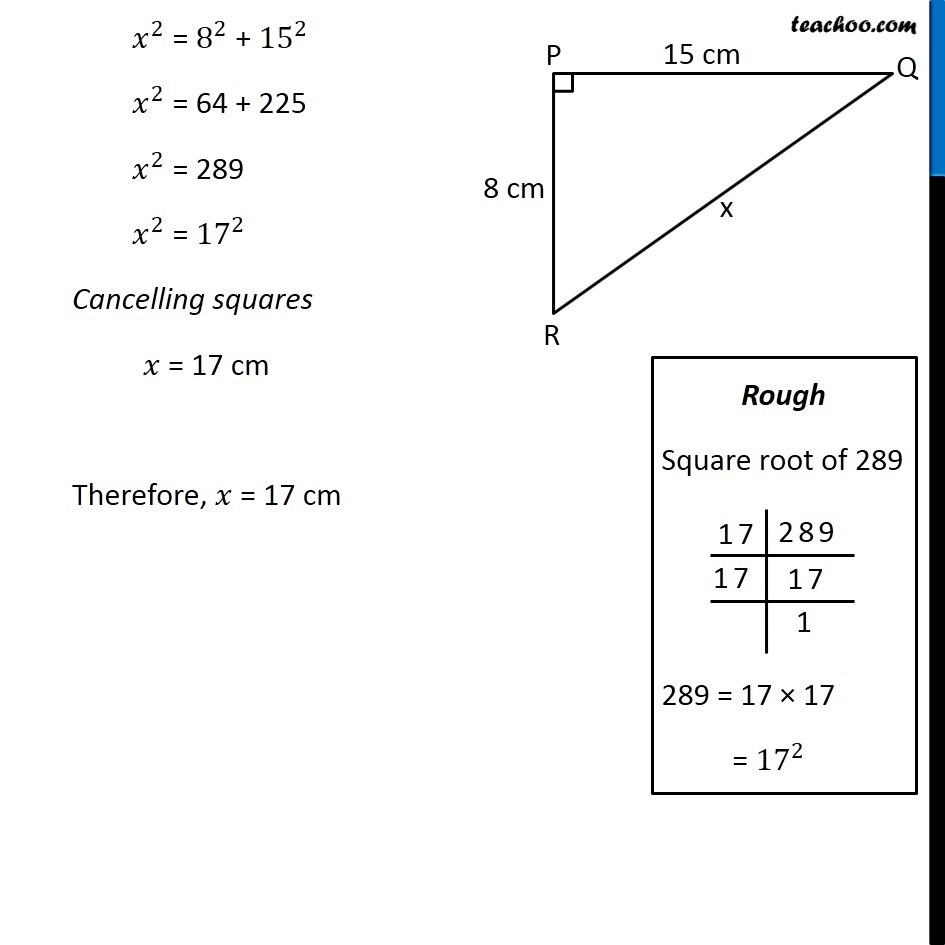Find x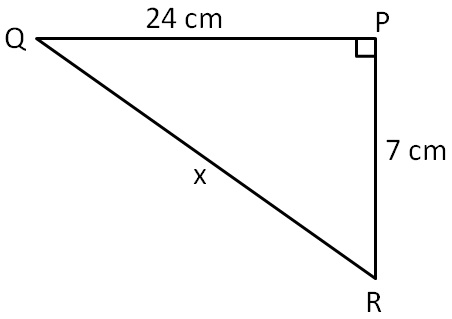Here,

∆PQR is a right angled triangle, right angled at P

And,

PQ = 24 cm, QR = x, PR = 7 cm

By Pythagoras Theorem

QR 2 = PQ 2 + PR 2

x 2 = (24) 2 + 7 2

x 2 = 576 + 49

x 2 = 625

x 2 = (25) 2

Cancelling squares

x = 25 cm

Therefore, x = 25 cm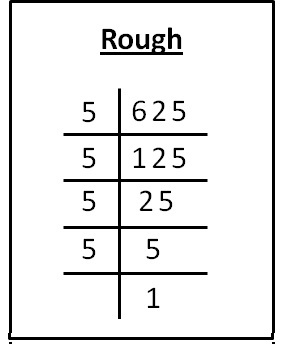625 = 5 × 5 × 5 × 5

= 25 × 25

= (25) 2

Find x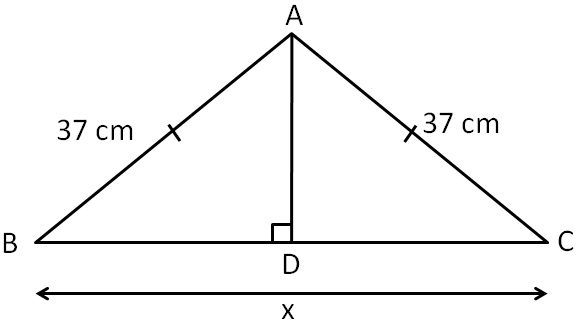In ∆ABC,

AB = AC

Hence, it is an isosceles triangle.

In isosceles triangle,

altitude and median are same

∴ AD is median of BC

i.e. D is mid-point of BC

∴ BD = DC = BC/2

BD = DC = x/2

In ∆ADC, right angled at D.

By Pythagoras Theorem

(AB) 2 = (AD) 2 + (BD) 2

(37) 2 + (12) 2 + (x/2) 2

1369 = 144 + x 2 /4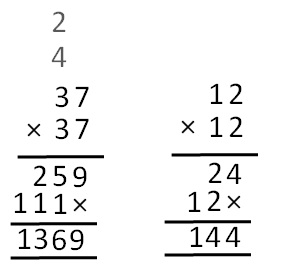1369 − 144 = x 2 /4

1225 = x 2 /4

1225 × 4 = x 2

4900 = x 2

x 2 = 4900

x 2 =  70 2

Cancelling squares

x = 70 cm

∴ x = 70 cm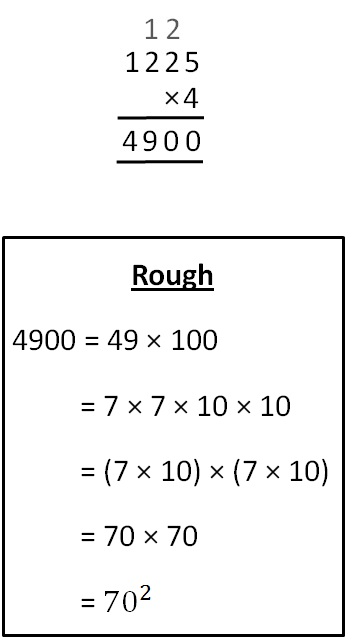Let's look at one last example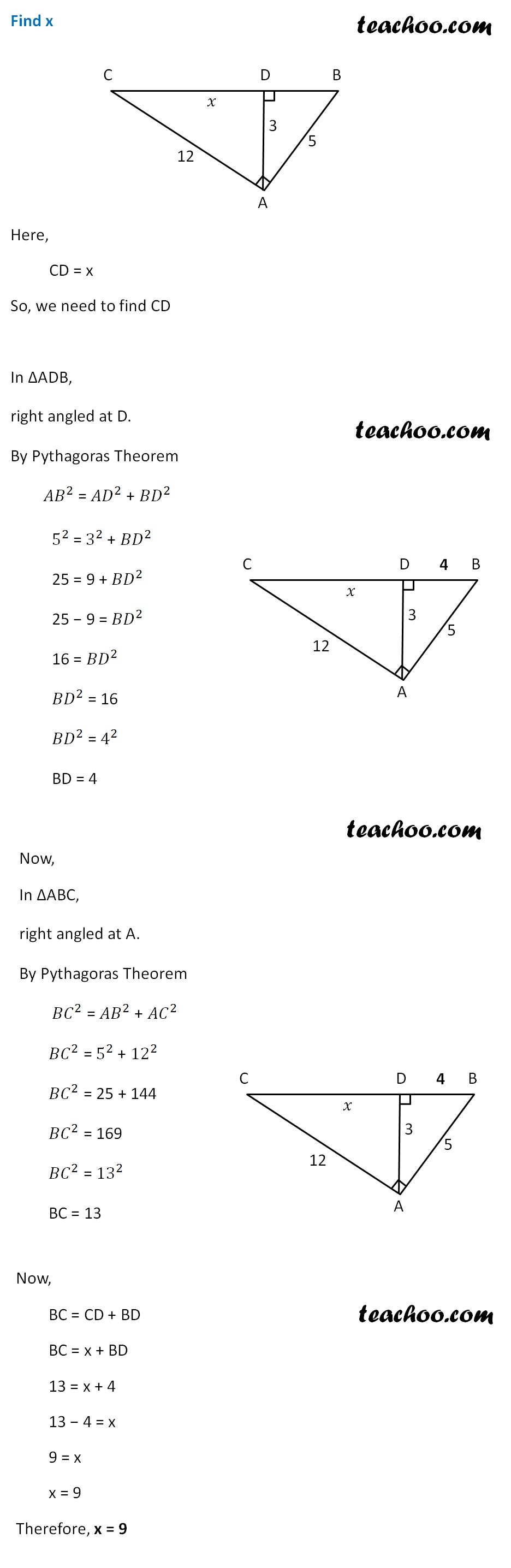Get live Maths 1-on-1 Classs - Class 6 to 12

### Transcript

∴ AD is median of BC i.e. D is mid-point of BC ∴ BD = DC = 𝐵𝐶/2 BD = DC = 𝑥/2 In ∆ADC, right angled at D. By Pythagoras Theorem 〖𝐴𝐵〗^2 = 〖𝐴𝐷〗^2 + 〖𝐵𝐷〗^2 〖(37)〗^2 + (12)^2 + (𝑥/2)^2 1369 = 144 + 𝑥^2/4 1369 − 144 = 𝑥^2/4 1225 = 𝑥^2/4 1225 × 4 = 𝑥^2 4900 = 𝑥^2 𝑥^2 = 4900 𝑥^2 = 702 Cancelling squares 𝑥 = 70 cm ∴ 𝑥 = 70 cm Rough 4900 = 49 × 100 = 7 × 7 × 10 × 10 = (7 × 10) × (7 × 10) = 70 × 70 = 〖70〗^2 Find x Here, CD = x So, we need to find CD In ∆ADB, right angled at D. By Pythagoras Theorem 〖𝐴𝐵〗^2 = 〖𝐴𝐷〗^2 + 〖𝐵𝐷〗^2 5^2 = 3^2 + 〖𝐵𝐷〗^2 25 = 9 + 〖𝐵𝐷〗^2 25 − 9 = 〖𝐵𝐷〗^2 16 = 〖𝐵𝐷〗^2 〖𝐵𝐷〗^2 = 16 〖𝐵𝐷〗^2 = 4^2 BD = 4 Now, In ∆ABC, right angled at A. By Pythagoras Theorem 〖𝐵𝐶〗^2 = 〖𝐴𝐵〗^2 + 〖𝐴𝐶〗^2 〖𝐵𝐶〗^2 = 5^2 + 〖12〗^2 〖𝐵𝐶〗^2 = 25 + 144 〖𝐵𝐶〗^2 = 169 〖𝐵𝐶〗^2 = 〖13〗^2 BC = 13 Now, BC = CD + BD BC = x + BD 13 = x + 4 13 − 4 = x 9 = x x = 9 Therefore, x = 9